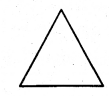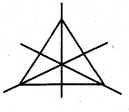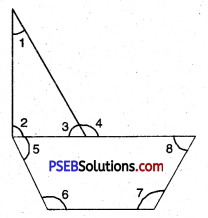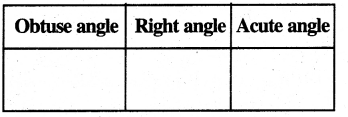# PSEB 5th Class Maths MCQ Chapter 7 Geometry

Punjab State Board PSEB 5th Class Maths Book Solutions Chapter 7 Geometry MCQ Questions and Answers.

## PSEB 5th Class Maths Chapter 7 Geometry MCQ Questions

Multiple Choice Questions

Question 1.
Name the angle marked on the cover of book.(a) Right angle
(b) Acute angle
(c) Obtuse angle
(d) Straight angle.
(a) Right angleQuestion 2.
The angle of 90° is called :
(a) Right angle
(b) Obtuse angle
(c) Acute angle
(d) Straight angle.
(a) Right angle

Question 3.
The angle greater than 90° is called:
(a) Acute angle
(b) Right angle
(c) Obtuse angle
(d) Straight angle.
(c) Obtuse angle

Question 4.
Make the axis of symmetry of the given figure.